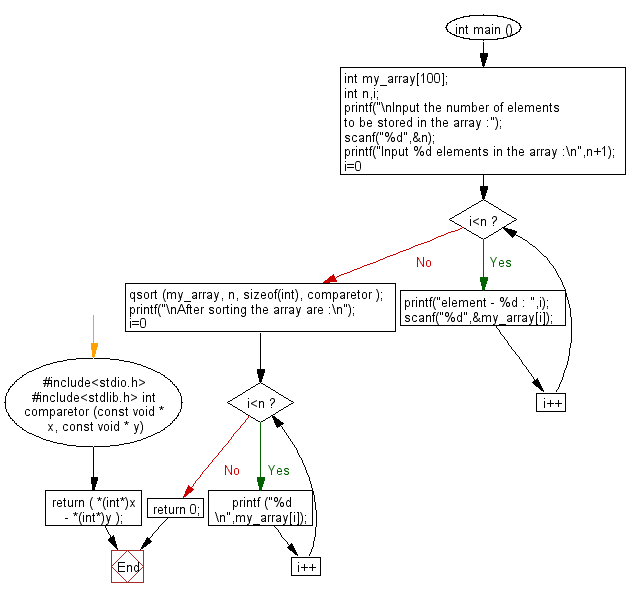﻿ C Program: Sort the elements of an array - w3resource# C Exercises: Sort the elements of an array

## C Variable Type : Exercise-6 with Solution

Write a C program to sort the elements of an array.

Sample Solution:

C Code:

``````#include<stdio.h>
#include<stdlib.h>

int comparetor (const void * x, const void * y)
{
return ( *(int*)x - *(int*)y );
}
int main ()
{
int my_array;
int n,i;
printf("\nInput the number of elements to be stored in the array :");
scanf("%d",&n);
printf("Input %d elements in the array :\n",n+1);
for(i=0;i<n;i++)
{
printf("element - %d : ",i);
scanf("%d",&my_array[i]);
}
qsort (my_array, n, sizeof(int), comparetor );
printf("\nAfter sorting the array are :\n");
for (i=0; i<n; i++)
printf ("%d \n",my_array[i]);
return 0;
}
```
```

Sample Output:

```Input the number of elements to be stored in the array :5
Input 6 elements in the array :
element - 0 : 2
element - 1 : 4
element - 2 : 3
element - 3 : 1
element - 4 : 5

After sorting the array are :
1
2
3
4
5
```

Flowchart:C Programming Code Editor:

Improve this sample solution and post your code through Disqus

What is the difficulty level of this exercise?

Test your Programming skills with w3resource's quiz.

﻿

## C Programming: Tips of the Day

How I can print to stderr in C?

The syntax is almost the same as printf. With printf you give the string format and its contents i.e.:

`printf("my %s has %d chars\n", "string format", 30);`

With fprintf it is the same, except now you are also specifying the place to print to:

```File *myFile;
...
fprintf( myFile, "my %s has %d chars\n", "string format", 30);```

`fprintf( stderr, "my %s has %d chars\n", "string format", 30);`